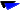# VACETS Regular Technical Column

"Science for Everyone"

"Science for Everyone" was a technical column posted regularly on the VACETS forum. The author of the following articles is Dr. Vo Ta Duc. For more publications produced by other VACETS  members, please visit the VACETS Member Publications page or Technical Columns page.

The VACETS Technical Column is contributed by various members , especially those of the VACETS Technical Affairs Committe. Articles are posted regulary on vacets@peak.org forum. Please send questions, comments and suggestions to vacets-ta@vacets.orgThu, 1 Dec 1994 Fermat's Last Theorem (2/2) Last month, we discussed the Fermat's Theorem and the prime number stuff and it was fun. Slowly, the discussion died out and was replaced with the voting issue. Sad, isn't it? Few days after the [SCIENCE FOR EVERYONE] FERMAT'S LAST THEOREM article was sent out last month, anh Phuong sent the news that the Fermat's last theorem problem was solved by Andrew Wiles and a colleague (I can not remember his name). What I heard at that time was that one of Wiles' colleague said that Wiles' new proof had no hole in it. I haven't heard any more news about that since. I'm wondering if Wiles' new proof has been published, or if the whole mathematical community has accepted the new proof, or if anyone has found new holes in Wiles' new proof. Do any of you hear any thing about that? In that [SCIENCE FOR EVERYONE] FERMAT'S LAST THEOREM article, I had three bonus questions and hoped that someone would be interested in the rewards (pho+? ta`u bay) and would answer them. I did not receive any answer to any of those three questions. It seems that many of us are more interested in politics than science. How sad! Is there a way to change that? Anyway, here are the three questions and the answers. Q1/ Find a counterexample to Fermat's Last Theorem. A1/ If Wiles' new proof is proved to be solid then the problem is solved. However, if it is not then shall we resume the discussion and try to solve it numerically? Q2/ a^n+b^n+c^n=c^n. It is trivial for n=1. For n=2 then 1^2+2^2+2^2=3^2. For n=3 then 3^3 + 4^3 + 5^3 = 6^3. Now try to find n>3 that would satisfy the equation. A2/ Yes, there are some n>3 that satisfy the equation. You probably can find them (using computer) in a second if you put your mind to it. Example: 95800^4 + 217519^4 + 414560^4 = 422481^4 2682440^4 + 15365639^4 + 18796760^4 = 20615673^4 Q3/ There are a^2+b^2=c^2 and a^3+b^3+c^3=d^3. Is there a^4+b^4+c^4+d^4=e^4? A3/ Yes. Ex: 30^4 + 120^4 + 272^4 + 315^4 = 353^4 And here is one involving with 5th power 27^5 + 84^5 + 110^5 + 133^5 = 144^5 Now, here is a new question for those who are interested in this matter. Is there any kind of relationship between Fermat's last theorem (a^n+b^n=c^n) and the other equations (a^n+b^n+c^n=d^n or a^n+b^n+c^n+d^n=e^n or...)? If there is, how do we find them and can we use them in proving or disproving Fermat's last theorem? Happy number crunching, everyone!!! Duc Ta Vo, Ph.D. ducvo@lanl.gov For discussion on this column, join vacets-tech@vacets.org Copyright © 1996 by VACETS and Duc Ta Vo : Other ArticlesHot Water Freezes Faster Than Cold ???Roots, Roots, and Roots!!!Fermat 's Last Theorem (1/2)Prime & FLTGreenhouse Effect: Atmospheric Trends of Greenhouse GasesFermat's Last Theorem (2/2)Greenhouse Effect: Carbon Removal and RecoveryBy Jove: Comet Crash PuzzlesCosmology: A Journey Through TimeThe Big BangDark MatterDark Matter (Part 2): WIMPs and other Exotic Particles.Spacetime-Travel and Relativity (Part 1)Spacetime-Travel and Relativity (Part 2)Lastest Known Prime Number Discovered Other LinksVACETS Home PageVACETS Electronic NewsletterVACETS FTP Site